## December 16, 2016

### Globular for Higher-Dimensional Knottings

#### Posted by John Baezguest post by Scott Carter

About 7 months ago, Jamie Vicary contacted me with a Globular worksheet of which, initially, I could make neither heads nor tails. He patiently explained to me that what I was looking at was an example that I had worked out for Bruce Bartlett one evening. He explained how to read it. Fast forward through a number of late night (for him) Skype sessions and a number of heartbreaking system errors for me, and now I feel that Globular is not only the best way to do higher dimensional knot theory and diagrammatic calculations, but it has the potential to be revolutionary. It will give insight into classical theorems and it will be used in the near future to create diagrammatic proofs of new theorems.

This first post will be about ordinary 3-dimensional knots.

The papers to read about Globular are Data structures for quasistrict higher categories by Jamie Vicary and Krzysztof Bar, and Globular: an online proof assistant for higher-dimensional rewriting in which Aleks Kissinger joins Jamie and Krzysztof to explain further and give some nice examples of Globular’s potential.

Most importantly, these worksheets are for unoriented knots and a knotted surfaces. Indeed, the hope here is to be able to address some key questions about non-orientable surfaces. If you want to create an oriented theory, then you need to add more objects and relations among them. I may create some of these soon. There will be differing color conventions within those worksheets. Color choices are helpful to the user to inform user of what will happen. Also, I am interested in knotted trivalent graphs and knotted foams. I have not yet created templates for their manipulations. To return to an old hobby, Globular is a lego set made of small pieces that you can create on your own, and here I am saying, “Hey, mommy look what I have done so far.”

The first worksheet to view is the classical knot template. Here we start in a 2-dimensional black ambient space. The first object that we see is a white dot. Next we create a cap:and a cup.The cap is, from my point of view, a $1$-morphism with source a pair of points and target the empty object. A cup has source and target reversed. The Reidemeister type II and type III moves are intrinsic to Globular, but the type I move has to be added. There are 6 variations of type I moves, and only two need to be added. In this worksheet, I have shown how two of the others follow from these two and the psy/ysp moves. The ysp moves, in whichgoes to,

are consequences of the categorical axioms. As an exercise, try and figure out what the remaining two type I moves are, and give proofs using Globular.

Also, zig-zag identities are not intrinsic. So left zig-zags and right zig-zags, and their inverses have been added.There is a learning curve to using Globular. You probably need a 3-button mouse and Google Chrome to optimize the experience. Within this template first click “allow undo” so this is turned off. Next left click on cell 2. A white dot appear. Click again and attach a second and third dot. Whether or not you attach above or below does not matter. Now click the identity button on the right. Move your mouse to the project box and change project to 1. Project is the number of dimensions you are suppressing. So at project 1 you are looking at a 2-dimensional projection of three straight arcs in 3-dimensional space. Let us braid as if they are locks of your little sister’s hair. Run your mouse to the bottom of the middle of the middle strand. Click left and swipe left. Go back to the middle; click left and swipe right. Continue at will. Now move your mouse pointer into the middle of one of the arcs. My favorite locale is at one of the inflection points. Click left and swipe left or right. Do this a few times, and see if you can create triangles at which the Reidemeister type III move (YBE) can occur.

To effect a Reidemeister type III move select (left-click) either the top-most or lower-most crossing among the three that define a potential move and that is either a top/middle or bottom/middle crossing. Then swipe the mouse in the direction of the move as if you are pinching the crossing between your fingers (cat’s cradle) and moving it.

As a final exercise with this template, go to Knot info, for example, and figure out how to construct the braid closer of each of the knots with fewer than, say 11 crossings. After having constructed a braid word (for example (1,1,1), hover the mouse to the left or right until you see the mouse-over say cell 1 @ [?,?], then left click to tensor with an identity string. Do so for as many strings as needed (in this case 2). Then left-click at the top/bottom middle string at which you want to create a cup or cap. A menu will pop-up with choices (in my case it says 1. cap 2. cap). Hover in the menu until a yellow rectangle shows up. The rectangle indicates where the cap will be attached. Do so until you have constructed the braid closer. Then use the classical knot template to demonstrate that each braid closure can be isotoped into its more standard view.

Posted at December 16, 2016 6:53 AM UTC

TrackBack URL for this Entry:   https://golem.ph.utexas.edu/cgi-bin/MT-3.0/dxy-tb.fcgi/2927

### Re: Globular for Higher-Dimensional Knottings

It’s great to see Globular starting to be used as a tool. If anyone hasn’t seen it in action, I recommend the video and other material here:

• Globular, Azimuth, December 14th, 2016.

The video is from Jamie’s talk at the workshop on ‘Compositionality’ at the Simons Institute for the Theory of Computing. Since composition is something category theorists know a thing or two about, there were a lot of such people at this workshop, and a lot of fun talks, and a lot of great conversations. I’ve been blogging about these talks on Azimuth, and you can reach those blog articles (and more videos) starting here:

Posted by: John Baez on December 16, 2016 7:28 AM | Permalink | Reply to this

### Re: Globular for Higher-Dimensional Knottings

At a conference over the summer, Jamie Vicary sat me down in front of Globular and constructed from scratch a rather complicated two-morphism I was thinking about. Having been somewhat sceptical up to the point, I was converted and spent some time getting my head round the interface. I have hopes that I can actually use it to prove a rather complicated identity that has eluded up to now, but unfortunately, teaching has intervened and I haven’t had chance to do it yet. But Globular definitely looks like it will be a useful tool.

Posted by: Simon Willerton on December 16, 2016 11:40 AM | Permalink | Reply to this

### Re: Globular for Higher-Dimensional Knottings

Scott, I realize you said

This first post will be about ordinary 3-dimensional knots.

but I didn’t see anything in this post about what you have done with Globular. So I chased down your page A collection of globular worksheets relating to knotted surfaces and braided manifolds which I guess contains some of the concrete examples you will be writing about in further posts.

Posted by: RodMcGuire on December 16, 2016 7:42 PM | Permalink | Reply to this

### Re: Globular for Higher-Dimensional Knottings

Rod, well my intension is to produce several worksheets and blog posts here about globular. For now, yes, you can go to this page and see some examples that I have produced. These include this sheet in which I demonstrate that globular can be used to demonstrate that the 1-twist spun trefoil is unknotted.

I also have this worksheet in which I give an explicit embedding in 6-space of the 3-fold branch cover of the 4-spher branched over the spun trefoil. This embedding fits into a tubular neighborhood of the standard 4-sphere in 6-space, and it is arranged in such a way that the projection from the tubular neighborhood onto its base space restricts to produce the branched covering space. but I haven’t yet advertised it since I thought I needed to give some background first.

I think that John has been busy elsewhere, and is not ready to release my next post. Meanwhile, I have been trying to write a third one. But for now, I welcome questions about those that you have viewed.

Posted by: Scott Carter on December 16, 2016 9:12 PM | Permalink | Reply to this

### Re: Globular for Higher-Dimensional Knottings

(For those for whom it is more convenient, a pdf of this post can be found here.)

Fantastic to see these posts! Apropos of not very much except the relevance of knot theory, I’d like to share some ideas I’ve been working on for a while. Any thoughts which anybody has would be most welcome. I’ve been kindly offered the possibility to write a guest post here about these ideas, but presenting the background in a pleasant way is a bit overwhelming. So instead I’m just going to present the ideas concisely here, and am happy to elaborate about anything.

What I’m going to talk about is to do with the Poincaré conjecture. The first reaction of any weather-beaten geometric topologist upon seeing these two words and seeing the simplicity and shortness of my arguments is probably going to be to dismiss them immediately! But I hope that one or two of you are generous and open-minded enough to have a think about them. Let me also try to bring things immediately down to earth. I did not set out to think about the Poincaré conjecture; I rather stumbled upon these ideas whilst discussing with a student, Reidun Persdatter Ødegaard, some work for her master thesis. If there is an error, there is an error; the ideas might be of some interest nevertheless. I would just ask that the argument be thought about on its own merits.

Enough of that! Let’s get down to it. I will first discuss what I like to call the ‘Poincaré conjecture for knots’. This is the following statement. Let $K$ be a framed knot (I will also denote by $K$ its associated blackboard framed knot diagram, and will switch between the two without mention). Let $M$ be the 3-manifold obtained by the integral Dehn surgery on $K$ determined by its framing. Then $M$ has trivial fundamental group if and only if $K$ is equivalent under the framed Reidemeister moves and Kirby moves to a $\pm 1$ framed unknot (a figure of eight).

What has this to do with the Poincaré conjecture? The latter says that any (closed) 3-manifold with trivial fundamental group is isomorphic to the 3-sphere. Any such 3-manifold is both connected and orientable. By a theorem of Lickorish and Wallace, for any (closed,) orientable, connected 3-manifold $M$, there is a framed link $L$ such that $M$ is isomorphic to the 3-manifold obtained by the integral Dehn surgery on $L$ determined by its framing. Moreover, by a theorem of Kirby, a pair of such 3-manifolds $M$ and $M'$ are isomorphic if and only if the corresponding blackboard framed link diagrams $L$ and $L'$ can be obtained from one another by a finite sequence of framed Reidemeister moves and Kirby moves. Accurately or not, I like to think of these two results together as analogous to the surgery proof of the classification of surfaces. Anyhow, by taking the integral Dehn surgery on a $\pm 1$-framed unknot with respect to its framing, we obtain the 3-sphere. Putting all this together, we see that the Poincaré conjecture can be reformulated as follows: if $M$ has trivial fundamental group, then the corresponding blackboard framed link diagram $L$ is equivalent under the framed Reidemeister moves and Kirby moves to the $\pm 1$-framed unknot. What I am calling the Poincaré conjecture for knots is the special case where $M$ comes from an $L$ that is a framed knot.

In fact, we can go further, and reformulate the Poincaré conjecture as a statement purely in diagrammatic knot theory. Indeed, given $M$ and $L$ as in the previous paragraph, the fundamental group of $M$ is isomorphic to $\pi_{1}(L)/\langle l \rangle$, the quotient of the fundamental group of $L$ by the normal subgroup generated by the longitude $l$ of $L$. And both $\pi_{1}(L)$ and $l$ can be defined from a link diagram. Thus the Poincaré conjecture becomes: if $L$ is a blackboard framed link diagram such that $\pi_{1}(L)/\langle l \rangle$ is trivial, then $L$ is equivalent under the framed Reidemeister moves and Kirby moves to a $\pm 1$-framed unknot.

So far, all of this is background, well-known to geometric topologists. What I am going to do first is to give an argument to prove this last statement when $K$ is a blackboard framed knot diagram. And in fact, I am going to argue that, in this special case, no Kirby moves are needed. That is to say, I am going to give an argument to show that if $K$ is a blackboard framed knot diagram such that $\pi_{1}(K)/\langle l \rangle$ is trivial, then $K$ is equivalent under the framed Reidemeister moves alone to a $\pm 1$-framed unknot. Thus we actually obtain something stronger than the Poincaré conjecture for knots: we also obtain the integral part of a theorem of Gordon and Luecke, that if we have a non-trivial (rational) Dehn surgery on a framed knot $K$ that gives the 3-sphere, then $K$ is isotopic to the unknot.

Begin, then, with a framed knot diagram $K$. Equip it with an orientation (any will do), which is the one we will use to define its fundamental group and longitude. Label the arcs of $K$. By a ‘word in the arcs of $K$’, I shall mean a monomial $a_{1}^{\pm 1} \cdots a_{n}^{\pm 1}$, where $a_{1}$, $\ldots$, $a_{n}$ are (labels of) arcs of $K$. I will say that a word $w$ in the arcs of $K$ is realisable if we can find a virtual knot $K_{w}$ which is equivalent to $K$ under the (virtual) framed Reidemeister moves, and which has the following property. First, if there are any occurrences of the longitude $l$ of $K$ as sub-words of $w$, then remove them all except one. For ease of notation, I will suppose that we have already done this for $w$. Then we ask that there is a point $p$ on $K_{w}$ such that as we walk around $K_{w}$ exactly once, in the direction defined by the orientation, beginning at $p$ and returning to $p$, then we pass successively and in order under the arcs $a_{1}$, \ldots, $a_{n}$, and under no other arcs; and the power of $a_{i}$ is the sign (the usual one defined for a crossing of an oriented link diagram) of the crossing where we pass under $a_{i}$.

Given a crossing $C$ of $K$ as follows (apologies for the ASCII figure, I hope that it displays correctly), I shall denote by $w_{C}$ the word $c^{-1}b^{-1}ab$ in the arcs of $K$.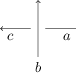Now, $\pi_{1}(K) / \langle l \rangle$ is isomorphic to the quotient of the free group $F(K)$ on the arcs of $K$ by the normal subgroup $N$ consisting exactly of words in the arcs of $K$ of the form $g^{-1}vg$ and their inverses, where $v$ is any concatenation of copies of $l$ and of words in the arcs of $K$ of the form $w_{C}$ for various crossings $C$ of $K$.

My key claim is that every word of $N$ of the form $g^{-1}vg$ which contains a copy of $l$ is realisable, and moreover, if a word $w$ is equivalent to such a word of $N$ under the equivalence relation of being able to add or delete pairs $aa^{-1}$ and $a^{-1}a$, where $a$ is an arc of $K$, then $w$ is also realisable.

Let us prove this. Take any $v$ as above. Since we delete all copies of $l$ from $v$ except one when defining realisability, and since I am assuming that there is at least one copy of $l$, we may assume that $v$ is of the form $w_{C_{1}} \cdots w_{C_{i}} \cdot l \cdot w_{C_{i+1}} \cdots w_{C_{n}}$ for some $n$. We begin at $p$. We will walk around $K$ in the same direction as our original orientation which we are using to define $\pi_{1}(K)$ and $l$. Suppose that $w_{C_{1}}$ looks as in the figure above. Take a small piece of the arc on which $p$ lies, just after $p$. Drag it, using only virtual R2 moves, so that it is near the above figure. Then slide it (using two R2 moves and an R3 move) under the above crossing, so that we have the following local picture. If the arc on which $p$ lies has label $d$, we label the arc indicated below of our new virtual knot diagram below by $d$ as well. In other words, we leave the labellings as they were, except that there is a ‘break’ in the arc labelled $d$.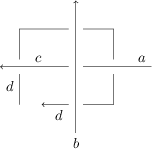We now proceed in exactly the same way for $w_{C_{2}}$, using the arc labelled $d$ in the above figure. And so on until we have done the same for $w_{C_{i}}$. At this point, we now encounter $l$ in our word. And we now walk all the way around the virtual knot which we have obtained so far, from where we were after carrying out the above procedure for $w_{C_{i}}$, stopping when we reach arc $d$, a little before we reach $p$. After this, we carry out the procedure above for $w_{C_{i+1}}$, \ldots, $w_{C_{n}}$, beginning with a little piece of arc between where we stopped and $p$. After we are finished with $w_{C_{n}}$, we simply walk to $p$.

Hopefully you agree that this realises our $v$!

Now, take the virtual knot $K_{v}$ that we have constructed to realise $v$. To realise $g^{-1}vg$, where $g$ is any arc of $K$, we proceed as follows. Take a small piece of the arc $g$. Using virtual R2 moves, drag it across $K_{v}$ so that it is near the point $p$. Then apply an R2 move so that we have the following local picture.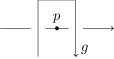Walking around our new virtual knot from $p$ in the same direction as before, we have realised $g^{-1}vg$.

To establish the claim, it remains to show that if we add or delete a pair $aa^{-1}$ or $a^{-1}a$, where $a$ is an arc of $K$, from such a $g^{-1}vg$, then $w$ is also realisable. To add a pair $aa^{-1}$ between say $b$ and $c$ in $g^{-1}vg$, then we apply the same idea that we have just seen: take a small piece of the arc labelled $a$ on $K_{g^{-1}vg}$, drag it using virtual R2 moves so that it is near $b$ and $c$, and then apply an R2 move so that we have the following picture.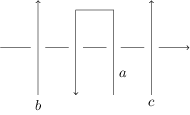The same argument works for adding $a^{-1}a$, just using the virtual R2 moves in a different way so that we can drag the arc $a$ over from the opposite side; and of course we could have $b^{-1}$ or $c^{-1}$ or both, and would be able to apply the same argument.

Suppose now that we wish to delete a pair $aa^{-1}$ from $K_{g^{-1}vg}$. Then we must successively walk first under $a$ and then under $a$ again in the opposite direction, without walking under any other arcs. This means that we have a local picture as follows, where there may be other arcs that pass under those shown or cross them virtually.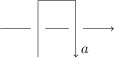Using R2 and R3 moves and their virtual counterparts, we can slide the arc $a$ over the other depicted arc, so that we have (exactly, if we zoom in closely enough) the following local picture.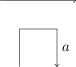If we now walk around our new virtual knot in exactly the same way as before, beginning and ending at $p$, we have cut out the $aa^{-1}$ pair. The same argument works for deleting a pair $a^{-1}a$.

Hopefully you agree that the claim has been demonstrated! Now, what can we do with it?

Suppose that $\pi_{1}(K) / \langle l \rangle$ is trivial. Then $N$ is $F(K)$. Let $a$ be an arc of $K$. Since $N$ is all of $F(K)$, we have that either $a$ or $a^{-1}$ is equal in $F(K)$ to a word of the form $g^{-1}vg$ for some $g$ and $v$, where $v$ is any concatenation of copies of $l$ and of words in the arcs of $K$ of the form $w_{C}$ for various crossings $C$ of $K$.

My second claim is that for at least one arc $a$ of $K$, the word $v$ in the word $g^{-1}vg$ which is equal to either $a$ or $a^{-1}$ contains at least one copy of $l$. For if this is not the case, then every arc of $K$ or its inverse is equal in $N$ to a $g^{-1}vg$ where $v$ is a product of $w_{C}$’s, that is to say, every arc of $K$ or its inverse is trivial in $\pi_{1}(K)$. But then $\pi_{1}(K)$ is trivial, and no knot has a trivial fundamental group.

Combining the first and the second claim, we obtain that, for at least one arc $a$ of $K$, we have that the word consisting just of $a$ or of $a^{-1}$ is realisable. That is to say, there is a virtual knot $K_{a}$ which is equivalent to $K$ under the (virtual) R2 and R3 Reidemeister moves, and in which there is a point $p$ of $K_{a}$ from which, when we walk around $K_{a}$ in a particular direction and return back to $p$, we pass only under a single arc, namely $a$.

Every virtual knot diagram with a single (classical) crossing of the kind we obtain by our methods is equivalent under virtual R2 and R3 moves to a classical $\pm 1$-framed unknot. Thus we deduce that $K$ is equivalent as a virtual knot under (virtual) R2 and R3 moves to a classical $\pm 1$-framed unknot. But if a pair of classical knots are equivalent as virtual knots using (virtual) R2 and R3 moves, then they are equivalent as classical knots under the framed Reidemeister moves (this is an almost immediate consequence of the fact that the fundamental rack is a complete invariant of classical framed knots). We conclude that $K$ is equivalent as a classical knot under the framed Reidemeister moves to a $\pm 1$-framed unknot, as required.

I would be delighted to hear what people think of this.

With some small adaptations, the same argument goes through for arbitrary links (not only knots) if a certain fact is true, to prove that if $\pi_{1}(L) / \langle l_1, \ldots, l_m \rangle$ is trivial, where the $l_i$’s are the longitudes of the components of $L$, then $L$ is equivalent under the framed Reidemeister moves and Kirby moves to the empty link. This is reformulation of the full Poincaré conjecture in diagrammatic knot theory. The Kirby moves are necessary here.

I will outline the necessary modifications. When defining realisability, we allow ourselves to delete copies of all longitudes, leaving only one copy of the longitude of one of the components, and no copies of the longitudes of any of the others. When constructing $L_{v}$, we must take $p$ to be a point of an arc of the component of $L$ from which the single longitude in $v$ comes. At the very end of the argument, we use $ab$, for a pair of arcs $a$ and $b$ of $L$, rather than $a$ for a single arc. (If there is only one arc of $L$, then the result is trivial.) We then obtain that $L$ must be equivalent under (virtual) R2 and R3 moves to a virtual link which looks locally as follows (or with $a$ and $b$ switched).Now, the classical Kirby moves allow us to delete the component consisting of the arc $a$. By induction on the number of components of $L$, we conclude that $L$ must be equivalent under virtual framed Reidemeister moves and classical Kirby moves to the empty link diagram.

Now, I expect to be able to conclude here that $L$ must then be equivalent under classical framed Reidemeister moves and the classical Kirby moves to the empty link diagram. If this is true, we are done. However, as far as I know, no proof has yet been given that if a classical framed link diagram is equivalent under virtual framed Reidemeister moves and classical Kirby moves to another classical framed link diagram, then they are in fact equivalent under classical framed Reidemeister moves and classical Kirby moves.

A couple of words on the use of virtual knot theory in my argument: it allows us to drag a piece of an arc to another part of the knot diagram without affecting realisability. It is very difficult to see how to do this in general using only classical moves. The theorem that classical knots which are equivalent virtually are in fact equivalent classically is deep, because although it is a simple consequence of the fact that the fundamental rack is a complete invariant, the latter is a deep theorem.

Finally, a lot of people have very generously taken time, in some cases a lot of it, to think about earlier incarnations of these ideas. I’d especially like to thank Tobias Barthel, Emily Riehl, and Louis Kauffman. Richard Garner, Bruno Martelli, Markus Szymik, and Reidun Persdatter Ødegaard also gave, at various stages, feedback for which I’m very grateful.

Posted by: Richard Williamson on December 23, 2016 12:02 PM | Permalink | Reply to this

Post a New Comment